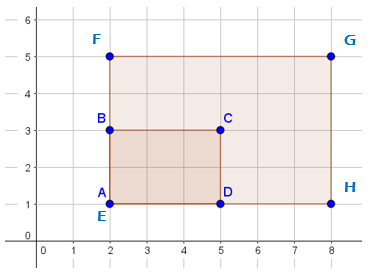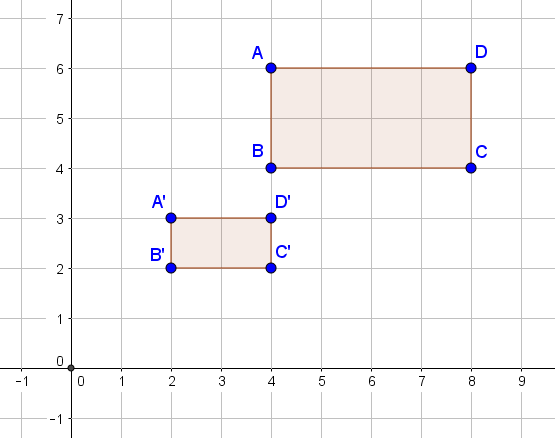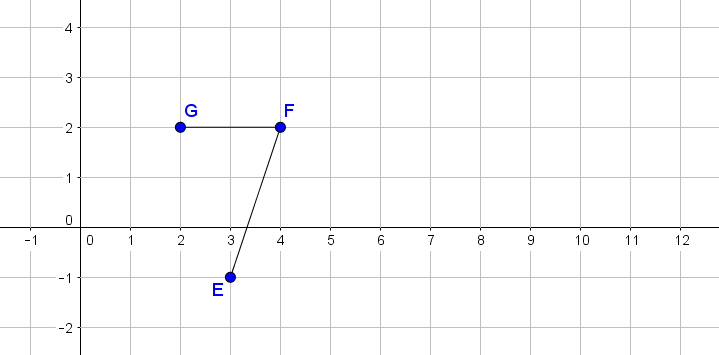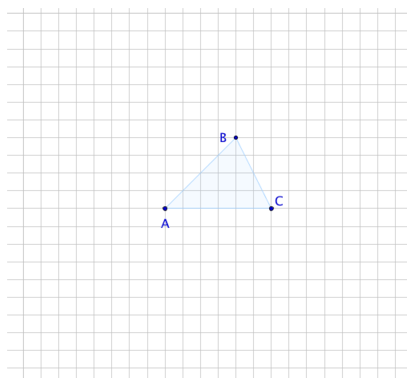# Transformations and Angle Relationships

## Objective

Describe and perform dilations.

## Common Core Standards

### Core Standards

?

• 8.G.A.4 — Understand that a two-dimensional figure is similar to another if the second can be obtained from the first by a sequence of rotations, reflections, translations, and dilations; given two similar two-dimensional figures, describe a sequence that exhibits the similarity between them.

?

• 7.G.A.1

• 7.RP.A.2

## Criteria for Success

?

1. Describe dilations using a center point of dilation and a scale factor.
2. Perform dilations using a center point of dilation on the origin and a center point of dilation on the figure.
3. Define similar figures as those that can be mapped to one another using dilations and other transformations.

## Tips for Teachers

?

• In this lesson, students work with dilations in isolation, distinguishing between points of origin on and off of the figure. In the next lessons, students will work with both dilations and rigid transformations.
• The following materials are useful for this lesson: graph paper and rulers.

#### Fishtank Plus

• Problem Set
• Student Handout Editor
• Vocabulary Package

## Anchor Problems

?

### Problem 1

Two diagrams below show a dilation of Rectangle ${ABCD}$ by a scale factor of 2.

If the scale factor is the same, then why do the two diagrams show different dilated figures?

Diagram 1:Diagram 2:### Problem 2

Rectangle ${ABCD}$ is similar to rectangle ${{A'B'C'D'}}$ because it can be dilated to map onto ${{A'B'C'D'}}$.

Describe the transformation. Include the scale factor and center point of dilation.### Problem 3

Angle ${EFG}$ is shown in the coordinate plane below.

Dilate the angle by a scale factor of 2 with the center of dilation at the origin.## Problem Set

?The following resources include problems and activities aligned to the objective of the lesson that can be used to create your own problem set.

• Examples where students describe dilations that are shown in the coordinate plane
• Examples where students perform dilations that are described
• Vary the types of examples to include centers at the origin and centers that are points on the figure, and to include scale factors greater than, less than, or equal to 1.
• Examples where students determine if two figures are similar (after a dilation); include examples and non-examples

?

Consider triangle ${ABC}$.1. Draw a dilation of ${ABC}$ with:

1. Center A and scale factor 2.
2. Center B and scale factor 3.
3. Center C and scale factor ${\frac{1}{2}}$

2. For each dilation, answer the following questions:

1. By what factor do the base and height of the triangle change?
2. By what factor does the area of the triangle change? Explain.
3. How do the angles of the scaled triangle compare to the original? Explain.

#### References

Illustrative Mathematics Effects of Dilations on Length, Area, and Angles

Effects of Dilations on Length, Area, and Angles, accessed on June 4, 2018, 1:48 p.m., is licensed by Illustrative Mathematics under either the CC BY 4.0 or CC BY-NC-SA 4.0. For further information, contact Illustrative Mathematics.

?